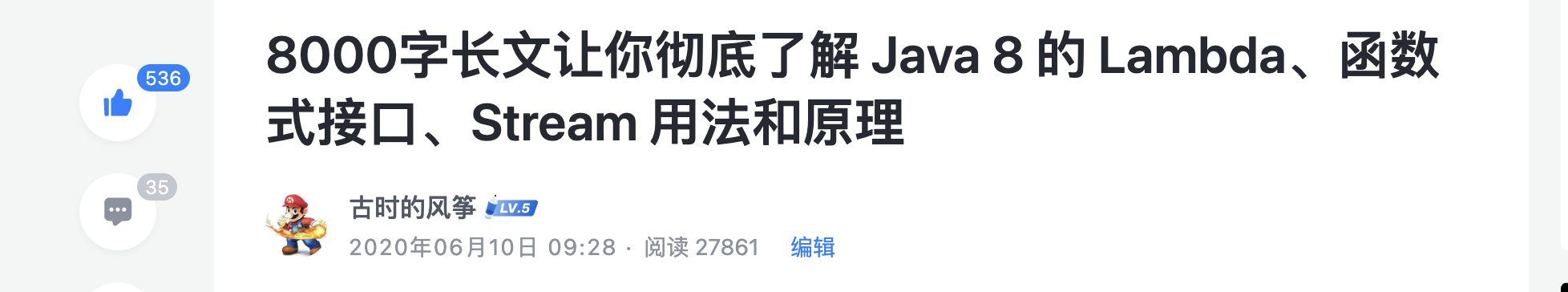# Java Stream 高级用法

Java Stream 是 JDK 8 开始提供的一种函数式风格的集合操作方法。我之前写过一篇 Java Stream 的文章 - 8000字长文让你彻底了解 Java 8 的 Lambda、函数式接口、Stream 用法和原理，在掘金社区获得了超过 500 个赞，说明大家还是很喜欢用 Stream 的。## 排序

### 基本数据类型排序

``````List<Integer> integerList = new ArrayList<>();
for (int i = 0; i < 5; i++) {
}
List<Integer> collect = integerList.stream()
.sorted()
.collect(Collectors.toList());
System.out.println(collect);
``````

``````List<Integer> integerList = new ArrayList<>();
for (int i = 0; i < 5; i++) {
}
List<Integer> collect2 = integerList.stream()
.sorted(Comparator.reverseOrder())
.collect(Collectors.toList());
System.out.println(collect2);
``````

`sorted()` 方法接收的参数是`Comparator` 函数式接口，在 8000字长文让你彻底了解 Java 8 的 Lambda、函数式接口、Stream 用法和原理 这篇文章清楚的讲了函数式接口和方法引用，可以翻过去看看。

### 非基本类型实体排序

``````import lombok.Data;

/**
* @author fengzheng
*/
@Data
public class Product {
/**
* 唯一标示
*/
private Integer id;

/**
* 所属类别
*/
private Integer type;

/**
* 商品名称
*/
private String name;

/**
* 价格
*/
private Double price;

}
``````

#### 按某一个字段排序

``````select * from product order by price asc
``````

``````List<Product> productList = initProductList();
List<Product> collect = productList.stream()
.sorted(Comparator.comparing(Product::getPrice))
.collect(Collectors.toList());
``````

``````List<Product> collect = productList.stream()
.sorted((x,y) -> x.getPrice().compareTo(y.getPrice()))
.collect(Collectors.toList());
``````

``````Comparator<Product> comparator = new Comparator<Product>() {
@Override
public int compare(Product p1, Product p2) {
return p1.getPrice().compareTo(p2.getPrice());
}
};

List<Product> collect = productList.stream()
.sorted((p1, p2) -> comparator.compare(p1, p2))
.collect(Collectors.toList());
``````

1. 前者小于后者，返回 -1；
2. 前者大于后者，返回 1；
3. 前者等于后者，返回 0；

``````List<Product> collect = productList.stream()
.sorted(Comparator.comparing(Product::getPrice).reversed())
.collect(Collectors.toList());
``````

``````List<Product> collect = productList.stream()
.sorted(Comparator.comparing(Product::getPrice,Comparator.reverseOrder()))
.collect(Collectors.toList());
``````

``````List<Product> collect = productList.stream()
.sorted((x,y) -> y.getPrice().compareTo(x.getPrice()))
.collect(Collectors.toList());
``````

``````Comparator<Product> comparator = new Comparator<Product>() {
@Override
public int compare(Product p1, Product p2) {
return p2.getPrice().compareTo(p1.getPrice());
}
};

List<Product> collect = productList.stream()
.sorted((p1, p2) -> comparator.compare(p1, p2))
.collect(Collectors.toList());
``````

#### 按照多个字段排序

``````select * from product order by type asc,price desc
``````

``````List<Product> collect = productList.stream().sorted(Comparator.comparing(Product::getType).thenComparing(Product::getPrice, Comparator.reverseOrder())).collect(Collectors.toList());
``````

``````Comparator<Product> typeComparator = new Comparator<Product>() {
@Override
public int compare(Product p1, Product p2) {
return p1.getType().compareTo(p2.getType());
}
};

Comparator<Product> priceComparator = new Comparator<Product>() {
@Override
public int compare(Product p1, Product p2) {
return p2.getPrice().compareTo(p1.getPrice());
}
};
List<Product> collect = productList.stream()
.sorted(typeComparator.thenComparing(priceComparator))
.collect(Collectors.toList());
``````

## 分组

### 根据 type 进行分组

``````select * from product group by type
``````

``````Map<Integer, List<Product>> map = productList.stream()
.collect(Collectors.groupingBy(Product::getType));
``````

``````Map<Integer, Long> map = productList.stream()
.collect(Collectors.groupingBy(Product::getType, Collectors.counting()));
``````

### 根据两个或多个字段分组

``````Map<String, List<Product>> map = productList.stream()
.collect(Collectors.groupingBy(p -> p.getType() + "|" + p.getPrice()));
``````

``````Map<String, List<Product>> map = productList.stream()
.collect(Collectors.groupingBy(p -> buildGroupKey(p)));

private static String buildGroupKey(Product p) {
return p.getType() + "|" + p.getPrice();
}
``````

### 嵌套分组

``````Map<Integer, Map<Double, List<Product>>> map = productList.stream()
.collect(Collectors.groupingBy(Product::getType, Collectors.groupingBy(Product::getPrice)));
``````

## teeing()

``````String result = productList.stream().collect(Collectors.teeing(
Collectors.minBy(Comparator.comparing(Product::getPrice)),
Collectors.maxBy(Comparator.comparing(Product::getPrice)),
(min, max) -> {
return min.get().getPrice() + "~" + max.get().getPrice();
}
));
System.out.println(result);
``````

``````4.347594572793579~89.43160979811124
``````

## 总结

Stream 提供了很丰富的 API ，最大的好处是让我们可以少写很多代码，熟练掌握之后，可以在一些对应的场景快速实现我们想要的逻辑。

## 文章目录

#### 相关文章

RPC框架的核心是什么？
0. Spring Cloud 是什么